﻿ 基于双电流互感器的电缆故障差动保护方法

# 基于双电流互感器的电缆故障差动保护方法Cable Fault Differential Current Protection Method Based on Dual Current Transformer

Abstract: As the urban construction process accelerates, the proportion of cable distribution in distribution networks is increasing, and the incidence of cable failures is also increasing. Due to the various forms of single-phase grounding faults, fault protection is greatly affected by factors such as transi-tion resistance, neutral grounding mode and current transformer imbalance. Fault troubleshooting is difficult, and cable trench explosions and other accidents occur frequently, which seriously af-fects the reliability of power supply. Therefore, it is necessary to study and improve the grounding fault protection method for distribution network. In this paper, the zero-sequence current distri-bution characteristics of single-phase grounding faults are analyzed in distribution network with neutral point ungrounded, arc-suppression coil grounded and small-resistance grounded. On this basis, considering the influence of transition resistance and the current transformer error, a cable fault differential current protection method based on dual current transformer is proposed. By in-stalling two sets of current transformers with different ranges at the two ends of the cable, the cur-rent transformer error is reduced to some extent, and the sensitivity of the differential protection is improved. The simulation results show that compared with the traditional single transformer, the cable fault differential current protection based on dual current transformer has higher re-sistance to transition resistance and sensitivity.

1. 引言

2. 配电网单相接地故障特征

2.1. 零序电流分布特征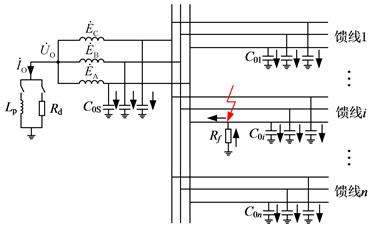Figure 1. Schematic diagram of single-phase grounding fault of distribution network

${\stackrel{˙}{I}}_{0j}=j\omega {C}_{0j}{\stackrel{˙}{U}}_{\text{O}}$ (1)

${\stackrel{˙}{I}}_{0i}=-\left[j\omega \left({C}_{0\Sigma }-{C}_{0i}\right){\stackrel{˙}{U}}_{\text{O}}+{\stackrel{˙}{I}}_{\text{O}}\right]$ (2)

${\stackrel{˙}{I}}_{\text{O}}=0$ (3)

${\stackrel{˙}{I}}_{\text{O}}=\frac{{\stackrel{˙}{U}}_{\text{O}}}{j\omega {L}_{\text{p}}}$ (4)

${\stackrel{˙}{I}}_{\text{O}}=\frac{{\stackrel{˙}{U}}_{\text{O}}}{{R}_{\text{d}}}$ (5)

2.2. 接地故障过渡电阻影响

${\stackrel{˙}{U}}_{\text{O}}\text{=}-\frac{{\stackrel{˙}{E}}_{A}}{1+j3\omega {C}_{0\Sigma }{R}_{f}}$ (6)

${\stackrel{˙}{U}}_{\text{O}}=\frac{-{\stackrel{˙}{E}}_{A}}{1+j3\omega {C}_{0\Sigma }{R}_{f}-j\frac{{R}_{f}}{\omega {L}_{p}}}$ (7)

${\stackrel{˙}{U}}_{\text{O}}=\frac{-{\stackrel{˙}{E}}_{A}}{1+j3\omega {C}_{0\Sigma }{R}_{f}+\frac{{R}_{f}}{{R}_{d}}}$ (8)

3. 电缆故障零序差动保护方法

3.1. 电流互感器误差影响

${\stackrel{˙}{I}}_{2}=\left({\stackrel{˙}{I}}_{1}-{\stackrel{˙}{I}}_{\mu }\right)/{n}_{\text{TA}}$ (9)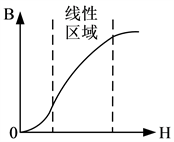Figure 2. Current transformer core magnetization curve

3.2. 基于双电流互感器的差动保护方法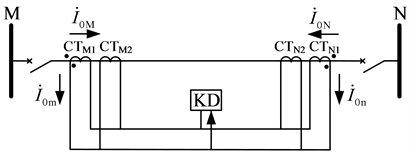Figure 3. Principle of differential current protection based on dual current transformers

${\stackrel{˙}{I}}_{\text{CD}}={\stackrel{˙}{I}}_{0M}+{\stackrel{˙}{I}}_{0N}$ (10)

${\stackrel{˙}{I}}_{\text{CD}}={\stackrel{˙}{I}}_{{C}_{0i}}$ (11)

${\stackrel{˙}{I}}_{\text{CD}}=-j\omega \left({C}_{0\Sigma }-{C}_{0i}\right){\stackrel{˙}{U}}_{\text{O}}$ (12)

${\stackrel{˙}{I}}_{\text{CD}}=-\left[j\omega \left({C}_{0\Sigma }-{C}_{0i}\right)+\frac{1}{j3\omega {L}_{\text{p}}}\right]{\stackrel{˙}{U}}_{\text{O}}$ (13)

${\stackrel{˙}{I}}_{\text{CD}}=-\left[j\omega \left({C}_{0\Sigma }-{C}_{0i}\right)+\frac{1}{3{R}_{\text{d}}}\right]{\stackrel{˙}{U}}_{\text{O}}$ (14)

${I}_{\text{ZD}}={K}_{\text{rel}}{I}_{\text{unb}}$ (15)

${I}_{\text{set}}=|j\omega \left({C}_{0\Sigma }-{C}_{0i}\right)\frac{{\stackrel{˙}{E}}_{A}}{1+j3\omega {C}_{0\Sigma }{R}_{f}}|$ (16)

${I}_{\text{set}}=|\left[j\omega \left({C}_{0\Sigma }-{C}_{0i}\right)+\frac{1}{j\omega {L}_{p}}\right]\frac{{\stackrel{˙}{E}}_{A}}{1+j3\omega {C}_{0\Sigma }{R}_{f}-j\frac{{R}_{f}}{\omega {L}_{p}}}|$ (17)

${I}_{\text{set}}=|\left[j\omega \left({C}_{0\Sigma }-{C}_{0i}\right)+\frac{1}{{R}_{d}}\right]\frac{{\stackrel{˙}{E}}_{A}}{1+j3\omega {C}_{0\Sigma }{R}_{f}+\frac{{R}_{f}}{{R}_{d}}}|$ (18)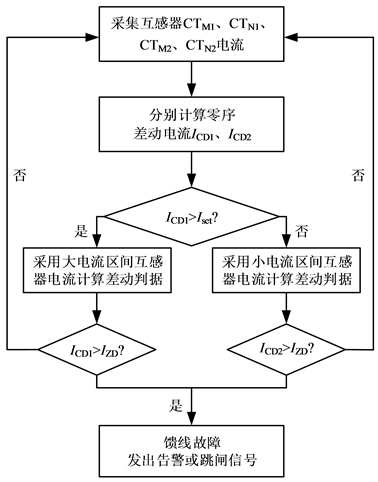Figure 4. Fault protection process based on dual current transformer

4. 仿真与分析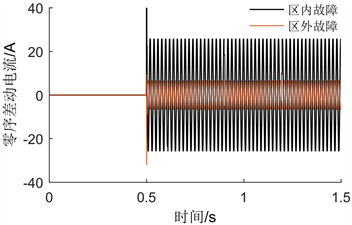Figure 5. Zero sequence differential current under internal and external faultsTable 2. The change of zero sequence differential current with transition resistance

5. 结论

 徐丙垠, 薛永端, 冯光, 王超. 配电网接地故障保护若干问题的探讨[J]. 电力系统自动化, 2019, 43(20): 1-7.

 龙毅, 欧阳金鑫, 熊小伏, 马格土, 杨明波. 基于零序功率变化量的配电网单相高阻接地保护[J]. 电工技术学报, 2019, 34(17): 3687-3695.

 李斌, 束洪春. 基于瞬时零序功率的谐振接地系统单相接地选线新方法[J]. 电力自动化设备, 2013, 33(4): 65-71+94.

 王福忠, 刘倩, 王要东, 周正彪. 基于小波理论和故障暂态信号的小电流接地故障选线策略[J]. 河南理工大学学报(自然科学版), 2017, 36(2): 105-110.

 吴乐鹏, 黄纯, 林达斌, 朱智军, 蒋洪涛. 基于暂态小波能量的小电流接地故障选线新方法[J]. 电力自动化设备, 2013, 33(5): 70-75.

 何瑞江, 胡志坚, 王天一. 计及分布式电源注入谐波的谐振接地系统故障选线方法[J]. 电网技术, 2019, 43(2): 670-680.

 孙其东, 张开如, 刘建, 伊利峰, 宋祥民, 李娅芸. 基于五次谐波和小波重构能量的配电网单相接地故障的选线方法研究[J]. 电测与仪表, 2016, 53(16): 1-4.

 田录林, 王伟博, 田琦, 罗燚, 张盛炜. 基于VMD能量相对熵的配电网单相接地故障选线方法[J]. 电气应用, 2019, 38(3): 47-53.

 王雪文, 石访, 张恒旭, 薛景润, 贠志皓, 谢伟, 凌平, 柳劲松. 基于暂态能量的小电流接地系统单相接地故障区段定位方法[J]. 电网技术, 2019, 43(3): 818-825.

 何连杰, 史常凯, 闫卓, 崔家瑞, 张波. 基于广义S变换能量相对熵的小电流接地系统故障区段定位方法[J]. 电工技术学报, 2017, 32(8): 274-280.

 贾文超, 黄少锋, 焦利霞. 双频注入式定子单相接地保护方案[J]. 电力自动化设备, 2014, 34(6): 158-161.

 孟安波, 葛佳菲, 李德强, 翁子豪, 焦夏楠. 基于纵横交叉算法的神经网络配电网故障选线研究[J]. 电力系统保护与控制, 2016, 44(21): 90-95.

 李玲玲, 李凤强, 王成山. 基于模糊综合决策的配电网单相接地故障选线[J]. 电力系统及其自动化学报, 2011, 23(5): 43-46.

 冯孝宏, 陈博博, 储亚男, 陈奎, 王爱东. 基于深度信息融合的小电流接地故障选线[J]. 电测与仪表, 2016, 53(20): 20-25.

 潘本仁, 管廷龙, 桂小智, 薛永端, 郭亮. 不接地系统高阻接地故障特征及选线适用性分析[J]. 电力系统及其自动化学报, 2017, 29(10): 52-59.

 李天友, 徐丙垠, 薛永端. 配电网高阻接地故障保护技术及其发展[J]. 供用电, 2018, 35(5): 2-6+24.

 徐玉琴, 杨浩, 李鹏. 不同接地方式电缆型配电网单相接地故障下的可靠性评估[J]. 电力系统保护与控制, 2018, 46(13): 41-47.

 张旭泽, 郑永康, 康小宁, 刘明忠, 孟雷, 陈迟. 智能变电站继电保护系统所面临的若干问题[J]. 电力系统保护与控制, 2018, 46(6): 90-96.

 李瑞生. 适用主动配电网的差动保护方案研究[J]. 电力系统保护与控制, 2015, 43(12): 104-109.

 范忻蓉, 张沛超, 方陈. 辐射型主动配电网的自适应方向保护方案[J]. 电力系统保护与控制, 2017, 45(18): 150-155.

Top